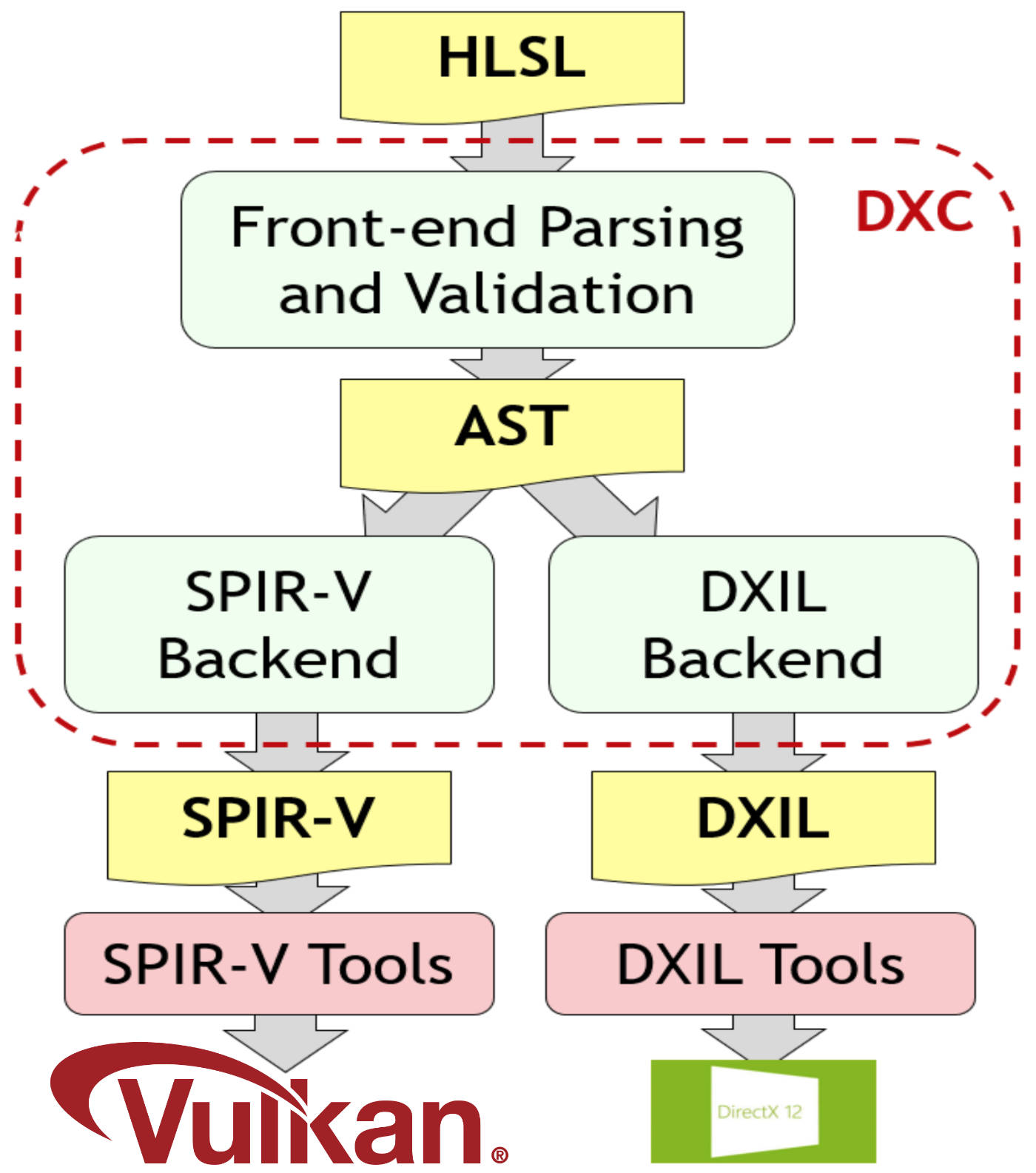# Shaders for Vulkan samples now also available in HLSL

One of Vulkan’s biggest additions (compared to OpenGL) was the introduction of the SPIR-V intermediate representation for shaders. This makes it possible to use different shader language front-ends for writing your shaders, with the only requirement being to be able to compile that language to valid SPIR-V with Vulkan semantics. Unlike OpenGL (except for GL_ARB_gl_spirv) this no longer confines you to use GLSL for writing Vulkan shaders.

And while GLSL is still the primary language for my C++ Vulkan samples, SPIR-V opens up options for different shader languages. One of those options is Microsoft’s HLSL which was initially developed for DirectX, but can now also compile to SPIR-V thanks to DXC’s SPIR-V codegen. Many developers prefer HLSL over GLSL both due to the language as well as the tooling for it.(Image courtesy of the Khronos Group)

A very detailed overview of how HLSL features map to SPIR-V is available here as part of the DXC repository.

And thanks to an external contribution by Ben Clayton from Google LLC, my Vulkan samples repository now also offers HLSL shaders versions for all the samples. He went through all the GLSL shaders and translated them to HLSL. There are a few caveats, but this readme has the important parts on the HLSL shaders and how to use them with my samples.

This should also be a great resource for those trying to get into HLSL, as the repository contains a wide range of shaders for all graphics and compute stages with differing complexities.

As an example here is a shader from the compute shader N-body particle simulation example:

GLSL:

// Copyright 2020 Sascha Willems

#version 450

struct Particle
{
vec4 pos;
vec4 vel;
};

// Binding 0 : Position storage buffer
layout(std140, binding = 0) buffer Pos
{
Particle particles[ ];
};

layout (local_size_x = 256) in;

layout (binding = 1) uniform UBO
{
float deltaT;
int particleCount;
} ubo;

layout (constant_id = 0) const int SHARED_DATA_SIZE = 512;
layout (constant_id = 1) const float GRAVITY = 0.002;
layout (constant_id = 2) const float POWER = 0.75;
layout (constant_id = 3) const float SOFTEN = 0.0075;

// Share data between computer shader invocations to speed up caluclations
shared vec4 sharedData[SHARED_DATA_SIZE];

void main()
{
// Current SSBO index
uint index = gl_GlobalInvocationID.x;
if (index >= ubo.particleCount)
return;

vec4 position = particles[index].pos;
vec4 velocity = particles[index].vel;
vec4 acceleration = vec4(0.0);

for (int i = 0; i < ubo.particleCount; i += SHARED_DATA_SIZE)
{
if (i + gl_LocalInvocationID.x < ubo.particleCount)
{
sharedData[gl_LocalInvocationID.x] = particles[i + gl_LocalInvocationID.x].pos;
}
else
{
sharedData[gl_LocalInvocationID.x] = vec4(0.0);
}

memoryBarrierShared();
barrier();

for (int j = 0; j < gl_WorkGroupSize.x; j++)
{
vec4 other = sharedData[j];
vec3 len = other.xyz - position.xyz;
acceleration.xyz += GRAVITY * len * other.w / pow(dot(len, len) + SOFTEN, POWER);
}

memoryBarrierShared();
barrier();
}

particles[index].vel.xyz += ubo.deltaT * acceleration.xyz;

particles[index].vel.w += 0.1 * ubo.deltaT;
if (particles[index].vel.w > 1.0)
particles[index].vel.w -= 1.0;
}

HLSL:

// Copyright 2020 Google LLC

struct Particle
{
float4 pos;
float4 vel;
};

// Binding 0 : Position storage buffer
RWStructuredBuffer<Particle> particles : register(u0);

struct UBO
{
float deltaT;
int particleCount;
};

cbuffer ubo : register(b1) { UBO ubo; }

#define MAX_SHARED_DATA_SIZE 1024
[[vk::constant_id(0)]] const int SHARED_DATA_SIZE = 512;
[[vk::constant_id(1)]] const float GRAVITY = 0.002;
[[vk::constant_id(2)]] const float POWER = 0.75;
[[vk::constant_id(3)]] const float SOFTEN = 0.0075;

// Share data between computer shader invocations to speed up caluclations
groupshared float4 sharedData[MAX_SHARED_DATA_SIZE];

{
// Current SSBO index
uint index = GlobalInvocationID.x;
if (index >= ubo.particleCount)
return;

float4 position = particles[index].pos;
float4 velocity = particles[index].vel;
float4 acceleration = float4(0, 0, 0, 0);

for (int i = 0; i < ubo.particleCount; i += SHARED_DATA_SIZE)
{
if (i + LocalInvocationID.x < ubo.particleCount)
{
sharedData[LocalInvocationID.x] = particles[i + LocalInvocationID.x].pos;
}
else
{
sharedData[LocalInvocationID.x] = float4(0, 0, 0, 0);
}

GroupMemoryBarrierWithGroupSync();

for (int j = 0; j < 256; j++)
{
float4 other = sharedData[j];
float3 len = other.xyz - position.xyz;
acceleration.xyz += GRAVITY * len * other.w / pow(dot(len, len) + SOFTEN, POWER);
}

GroupMemoryBarrierWithGroupSync();
}

particles[index].vel.xyz += ubo.deltaT * acceleration.xyz;

}